Electricity & Megnetism

# Energy stored in capacitors

The electrical energy stored in capacitors comes from the electric field that is established between their plates. This energy, of a potential and electrical nature, is numerically equal to the work required to charge the two capacitor plates and is also directly proportional to both the amount of stored electrical charges and the electrical potential difference between their armatures.

## Capacitance

Capacitance is the quantity that measures the amount of charge that a capacitor is able to store for each volt of potential difference that is applied between its plates. The unit of measurement for capacitance is the farad (F).

The formula used to calculate capacitance is as follows: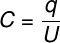C – capacitance (F)

Q – stored charge (C)

U – potential difference (V)

## Energy stored in capacitors

As the capacitor is charged, the potential difference between its plates increases, as shown in the following graph: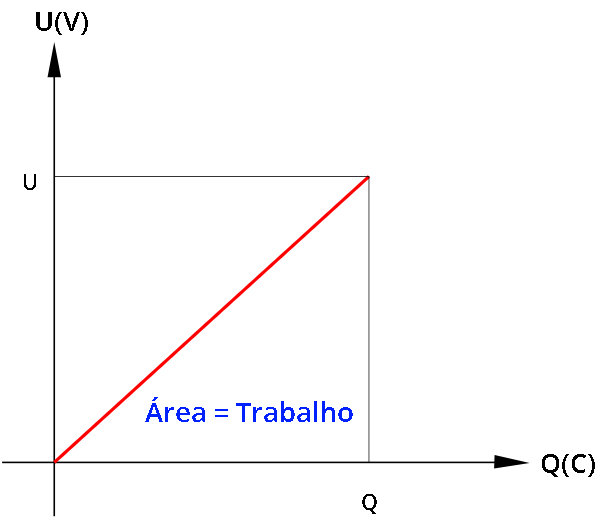In the graph, the area below the line represents the work required to charge the capacitor. This work, in turn, corresponds to all the energy stored in the capacitor. According to the chart format, the area to be calculated is that of a right triangle .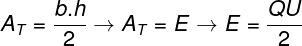Formulas for energy stored in capacitors

In addition to the classic formula used to calculate the energy stored in capacitors from the area of ​​the UxQ graph, there are other formulas that allow us to calculate such energy. These formulas can be obtained if we change the variables of the formula shown above. Watch: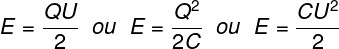## Solved exercises on energy in capacitors

Question 1 — (PUC – PR) Ventricular fibrillation is a process of disordered contraction of the heart that leads to a lack of blood circulation in the body, called cardiorespiratory arrest. A cardiac defibrillator is a device that applies a pulse of electrical current through the heart to restore heart rhythm. The equipment is basically a charging and discharging circuit of a capacitor (or capacitor bank). Depending on the characteristics of the emergency, the doctor controls the electrical energy stored in the capacitor within a range of 5 to 360 J. Suppose the graph given shows the charge curve of a capacitor of a defibrillator. The equipment is set to charge the capacitor across a potential difference of 4 kV What is the level of energy accumulated in the capacitor that the doctor has adjusted?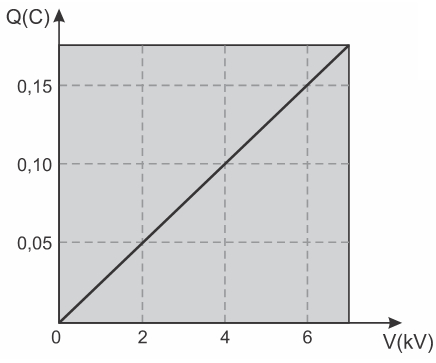a) 100 J

b) 150 J

c) 200 J

d) 300 J

e) 400 J

Resolution:

The energy stored in the capacitor can be easily calculated if we know the magnitude of the charge and the potential difference between its plates.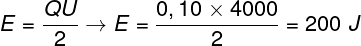Based on the calculation performed above, we find that the energy stored in the capacitor is equal to 200 J, so the correct alternative is the letter C .

Question 2 — A parallel plate capacitor is charged with an electric charge of 0.5 nC when connected to a battery of electromotive force equal to 20 V. Determine the magnitude of the electric potential energy stored in this capacitor and mark the correct alternative .

a) 2.5.10 -9 J

b) 3.10 9 J

c) 5.10 -9 J

d) 0.25.10 -9 J

Resolution:

Let’s calculate the energy stored in the capacitor. For this, we will use the formula where we multiply the electrical voltage by the stored charge and divide the result by two. Watch: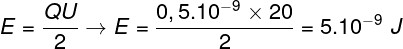Based on the calculation, the correct answer is the letter C.